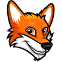## Python maintainability best practice

Code Review Doctor suggests a fix if your code does not follows Python maintainability best practices.

This frees your team to be more agile - they can focus on adding value rather than fighting with old tech debt.code-review-doctorbotsuggested changes just now
helpers.py
 `1` `+` `class FooBarClass(object):`

A class is inherited from `object` by default, so explicitly inheriting from object is redundant. Removing it keeps the code simpler.

Suggested changes
 `-` `class FooBarClass(object):` `+` `class FooBarClass:`
Commit suggestion
 `2` `+` ` pass`
validation.py
 `1` `+` `if isinstance(item, int) or isinstance(item, float):`

`isinstance` can take multiple types, so there is no need to call them multiple times for each type.

Suggested changes
 `-` `if isinstance(item, int) or isinstance(item, float):` `+` `if isinstance(item, (int, float)):`
Commit suggestion
 `2` `+` ` pass`
validation.py
 `1` `+` `if isinstance(item, int) or isinstance(item, float):`

`isinstance` can take multiple types, so there is no need to call them multiple times for each type.

Suggested changes
 `-` `if isinstance(item, int) or isinstance(item, float):` `+` `if isinstance(item, (int, float)):`
Commit suggestion
 `2` `+` ` pass`
models.py
 `1` `+` `class FooBarClass(NamedTuple):`

Consider using the `dataclass` here instead for simplicity, performance gains, and consistency

Suggested changes
 `-` `class FooBarClass(NamedTuple):` `+` `@dataclass(frozen=True)` `+` `class FooBarClass:` ` pass`
Commit suggestion
 `2` `+` ` pass`
helpers.py
 `1` `+` `class FooBarClass(Bar):` `2` `+` ` def foo(self, bar):` `3` `+` ` super(Foo, self).foo(bar)`

It's unnecessary to use arguments when calling super for the parent class.

Suggested changes
 `-` ` super(Foo, self).foo(bar)` `+` ` super().foo(bar)`
Commit suggestion
helpers.py
 `1` `+` `value = f'some-value'`

f-string is unnecessary here as there are no placeholders in the string.

Suggested changes
 `-` `value = f'some-value'` `+` `value = 'some-value'`
Commit suggestion
helpers.py
 `1` `+` `foo = lambda x: bar(x)`

Avoid unnecessarily wrapping a function in a lambda

Suggested changes
 `-` `foo = lambda x: bar(x)` `+` `foo = bar`
Commit suggestion
helpers.py
 `1` `+` `any([item for item in items])`

`all` and `any` can take a generator, so constructing a list first may be unnecessary.

Suggested changes
 `-` `any([item for item in items])` `+` `any(item for item in items)`
Commit suggestion
helpers.py
 `1` `+` `def foo_bar() -> "FooBarClass":`

Use type identifiers instead of using string type hints.

Suggested changes
 `-` `def foo_bar() -> "FooBarClass":` `+` `def foo_bar() -> FooBarClass:` `Expand 4 lines ...`
Commit suggestion
 `2` `+` ` return FooBarClass()` `3` `+` ` ` `4` `+` `class FooBarClass:` `5` `+` ` pass`
helpers.py
 `1` `+` `def foo_bar() -> Union[int, None]:`

This could be simplified by using `typing.Optional`

Suggested changes
 `-` `def foo_bar() -> Union[int, None]:` `+` `def foo_bar() -> Optional[int]:`
Commit suggestion
 `2` `+` ` # sometimes return int other times None`
helpers.py
 `1` `+` `dict((item, get_value(item)) for item in items)`

Using list and dict comprehension is simpler and computationally quicker than calling `list()` and `dict()`.

Suggested changes
 `-` `dict((item, get_value(item)) for item in items)` `+` `{item: get_value(item) item item in items}`
Commit suggestion
helpers.py
 `1` `+` `values = list([1, 2, 3])`

Using list/set/dict literal syntax is simpler and computationally quicker than calling `list()`, `set()`, or `dict()`.

Suggested changes
 `-` `values = list([1, 2, 3])` `+` `values = [1, 2, 3]`
Commit suggestion
helpers.py
 `1` `+` `def get_name(user):` `2` `+` ` return "%s %s" % (user.first_name, user.last_name)`

f-string is easier to read, write, and less computationally expensive than legacy string formatting.

Suggested changes
 `-` ` return "%s %s" % (user.first_name, user.last_name)` `+` ` return f'{user.first_name} {user.last_name}'`
Commit suggestion
helpers.py
 `1` `+` `from typing import Optional` `2` `+` ` ` `3` `+` `def foo_bar(value: Optional[str] = None):` `4` `+` ` if value:`

Checking Optional variables against `None` would be more explicit.

Suggested changes
 `-` ` if value:` `+` ` if value is not None:`
Commit suggestion
 `5` `+` ` ...`
catalogue.py
 `1` `+` `with open('some/path.json') as f:` `2` `+` ` content = json.loads(f.read())`

`json.load(f)` simplifies reading JSON from disk.

Suggested changes
 `-` ` content = json.loads(f.read())` `+` ` content = json.load(f)`
Commit suggestion
catalogue.py
 `1` `+` `with open('some/path.json', 'w') as f:` `2` `+` ` f.write(json.dumps({'foo': 'bar'}))`

`json.dump(f)` simplifies writing JSON to disk.

 `-` ` f.write(json.dumps({'foo': 'bar'}))` `+` ` json.dump({'foo': 'bar'}, f)`Update catalogue.py